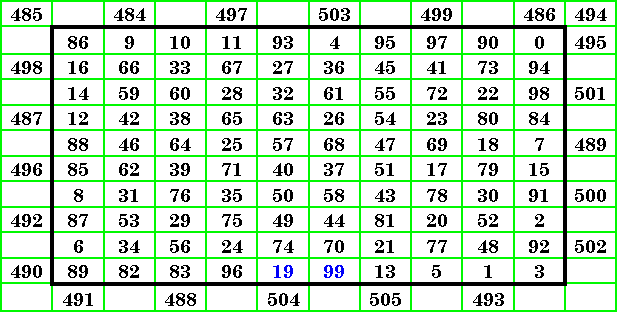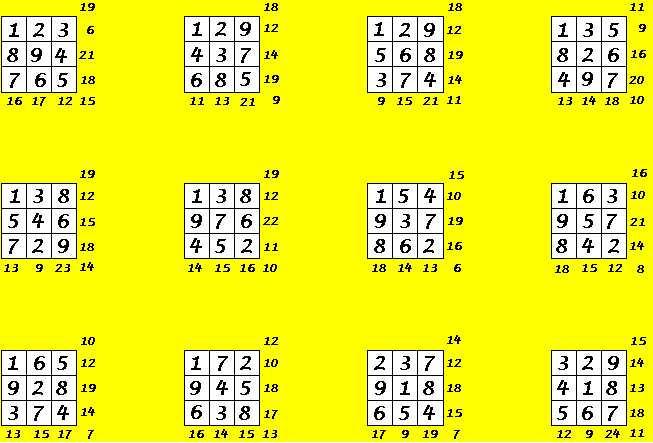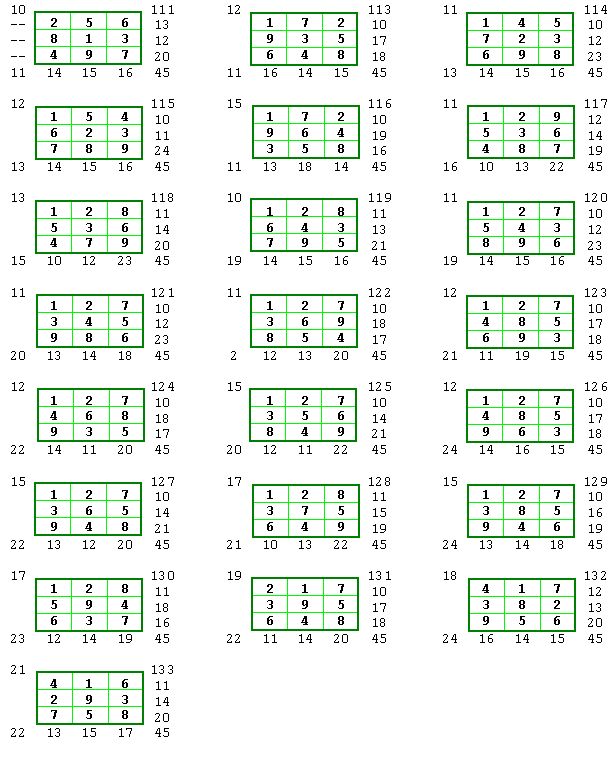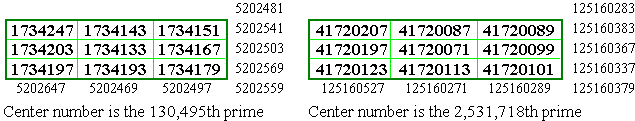# Anti-magic Squares

A pattern closely related to magic squares but relatively unexplored, is the anti-magic square.

Here we present some examples of them and their sibling, the heterosquare.

Heterosquare: similar to a magic square except all rows, columns and main diagonals have different sums.
Anti-magic Square: similar to a heterosquare except all rows, columns and main diagonals have consecutive sums.

### Antimagic Squares

Orders 4 to 9. Rows, columns and diagonals sum different but are consecutive.

### Order-10 Anti-Magic Squares

This special anti-magic square has the numbers 19 & 99 in the central bottom cells.

### Order-3 Heterosquares

12 different squares. Each of the 8 lines has a different sum. Also orders 4, 5, 6..

### Peter Bartsch's Heterosquares

This material added March 28/03. Real and prime heterosquares.

### Enoch Haga’s Prime Heterosquares

The rarity of these special patterns may surprise you (May, 2004).

### Anurag Sahay's Prime Heterosquares

Download an Excel spreadsheet listing many heterosquare and anti-magic squares.### Antimagic Squares

Anti-magic squares are a subset of heterosquares were the row, column, and diagonal sums are consecutive integers. These six use the consecutive digits from 1 to n2. Because they are anti-magic squares, the sums are consecutive numbers.

They are much more difficult to construct then heterosquares. There are no order 3 anti-magic squares. As the order increases, construction becomes easier , although there doesn't seem to be any standard routine available for constructing them.

Addendum: John Cormie developed several methods in 1999 for generating antimagic squares. See note in next section.
J. Lindon introduced the subject and published orders 4 to 9 antimagic squares (different then those shown here) in 1962.

 Order-8 S = 251 - 268 260 Order-7 S = 167 - 182 175 9 41 37 46 55 15 49 16 268 19 8 32 18 22 48 35 182 60 10 27 21 50 54 22 23 267 11 33 10 30 43 15 27 169 2 59 28 56 19 17 44 40 265 Order-4 29 - 38 34 46 9 13 14 17 23 49 171 64 13 35 14 25 57 18 36 262 2 15 5 13 35 40 45 39 12 1 4 31 172 3 63 31 45 42 11 43 20 258 16 3 7 12 38 20 2 26 42 38 41 5 174 52 4 39 24 32 47 6 51 255 9 8 14 1 32 7 34 37 25 44 24 6 177 30 62 1 38 33 7 53 29 253 6 4 11 10 31 36 47 16 29 3 21 28 180 34 5 61 12 8 58 26 48 252 33 30 37 36 29 179 178 173 170 168 176 181 167 ... 254 257 259 256 264 266 261 263 251 Order-9 S = 359 - 378 369 6 51 40 67 53 60 1 63 30 371 66 49 57 3 28 59 15 72 21 370 Order-6 S = 104 - 117 111 7 35 31 81 44 58 11 73 25 365 Order-5 S = 60 - 71 65 3 18 36 17 15 27 116 70 55 69 5 16 13 75 4 56 363 5 8 20 9 22 64 23 32 6 10 30 12 113 18 27 33 65 45 62 8 61 43 362 19 23 13 10 2 67 35 1 14 19 34 5 108 42 41 9 48 32 20 78 17 74 361 21 6 3 15 25 70 33 25 4 11 13 24 110 76 38 47 10 68 19 80 14 26 378 11 18 7 24 1 61 2 22 20 26 16 21 107 24 39 36 79 46 50 22 52 29 377 12 14 17 4 16 63 9 8 29 31 7 28 112 64 37 54 2 34 23 77 12 71 374 68 69 60 62 66 71 105 106 109 114 115 117 104 373 372 376 360 366 364 367 368 375 359### A Special  Order-10 Antimagic SquareA present day Anti-Magic analogue to Durer's 1514 magic square. This one for the year 1999.

This square appears on the Table of Contents page of John Cormie and Václav Linek ’s excellent Antimagic Squares site.
Notice that the 22 line, column and diagonal totals are the consecutive numbers from 484 to 505.### Order-3 Heterosquares

A heterosquare was defined in Mathematics Magazine, 1951, as an x by x array of integers from 1 to n2 such that all rows, columns and main diagonals have different sums.

In these twelve 3 x 3 heterosquares, the 3 rows, 3 columns, and 2 diagonals all have unique arrangements of different sums.
Are there any other basic arrangements?Examples of order 4, 5, and 6 heterosquares (one of the many possible for each order).The even orders are simply constructed by entering the numbers in order, then changing the positions of numbers 1 and 2.

The order 5 (or any odd order) is constructed by entering the numbers in order in a spiral starting with the center cell (or a corner cell).

References.
J. A. Lindon, Anti-magic Squares, Recreational Mathematics Magazine, No. 7, Feb. 1962, pp16-19
J. S. Madachy, Mathematics on Vacation, Thomas Nelson & Sons, 1996, pp 101-110
John Cormie and Václav Linek ’s excellent Antimagic Squares site. Sorry, this site is no longer available. Try http://io.uwinnipeg.ca/~vlinek/jcormie/ at http://web.archive.org/### Peter Bartsch's Heterosquares

Introduction

Are there other basic arrangements?

The question I asked originally when I presented the 12 heterosquares above was answered by Peter Bartsch, in correspondence starting in Nov., 2002.
The following is condensed from material supplied by him.

He reports that there are 3120 different basic (no rotations or reflections) heterosquares of order 3 using the numbers 1 to 9.

He coined the term ‘real’ for those heteroquares that consist of 17 distinct numbers, 9 for the interior numbers and 8 for the line sums. It turns out there are only 760 real heterosquares using the integers 1 to 9. Only two of the 12 heterosquares I show above are ‘real’!

The following 22 order 3 heteroquares are from the list of 760 real order 3 heteroquares. Each has a different grand total, which is the sum of the 9 interior numbers and the 8 line sum numbers. These totals range between 111 and 133, with none totalling 112.

The line sums contain anywhere from 2 to 7 consecutive numbers. None have all eight sums consecutive. They would then be antimagic squares, and there are no antimagic squares of order 3!Summary

There are 3120 basic heterosquares (HS) of order 3 using the numbers 1 to 9. Of these, 760 are real HS.
414 basic HS have two consecutive numbers in the sums. 1352 have 3 consecutive numbers, 816 have 4 consecutive numbers, 374 have 5, 126 have 6, and 38 HS have 7 sums in consecutive order.

### Prime Heterosquares

This material was supplied by Peter Bartsch between  November 2002 and March 2003.
Note that the first two are by Carlos Rivera , the third one by Jean-Charles Meyrignac.
Meyrignac's solution is believed to be the smallest possible order 3 prime heterosquare.Problem 69 of Carlos Rivera's http://www.primepuzzles.net/The following six heterosquares use the 9 consecutive primes from 31 to 67, so the total of these 9 cells is always 439 (a prime).

The 8 line sums are all primes in the range from 109 to 181.
Their sums form the totals, 1621, 1637, 1653, 1621, 1583, 1601. All are prime except 1653.
The squares are displayed and arranged according to Frenicle's index method.
Actually, Peter had sent many more examples, but all proved to be disguised versions of these six.Contact Peter Bartsch at pbartsch@synstar.de
See my page on Prime magic squares.### Enoch Haga’s Prime Heteromagic Squares

 On May 3, 2004, I received from Enoch Haga, two order 3 heteromagic squares consisting of prime numbers. Enoch has adopted a slightly different approach. He is searching for 9 consecutive primes that form a heterosquare when placed in order in this spiral pattern. He also requires that all 8 sums also be prime numbers. Because all 17 primes are distinct numbers, these are ‘real’ heterosquares by Peter Bartsch’s definition.As expected, these patterns are quite rare. However, it may come as a surprise to see just how rare they are!
In Enoch’s search, he keeps a running count of the groups of 9 primes that he checks.
The primes from 2 to 23 is count 1, 3 to 29 is count 2, etc.

The first group of 9 consecutive primes that form a heterosquare when placed in order in the above pattern occurs at count 130,495!
Another way to say this is that 1,734,133 is the 130,495th prime.For those that are interested, Enoch has provided a Ubasic listing of a  program to find the second solution.On May 6, 2004 I received another email from Enoch. I quote.

 You may be interested in this new kind of heterosquare. I am using the spiral and the count. I call this new kind of heterosquare a Ring Heterosquare. Here's how it works: The center of the square may be called the hub. This is prime A. All succeeding primes must be consecutive and follow a spiral pattern. The sums for a 3 x 3 square are calculated as follows, and each must be prime: Horizontal row 1, Vertical column 1, Horizontal row 3, Vertical column 3. These four form a box around the hub. Then the main diagonals are calculated, forming two more sums. Finally the value of the hub, the 2 horizontals, the 2 verticals, and 2 main diagonals are added, and the sum itself must be prime. All sums must be distinct.These are the first two 'ring' prime heterosquares. (Click image to enlarge).

Actually, because no primality test is made for the sums of the middle row and column, these squares are not necessarily PRIME heterosquares.
In both of these examples, both of these sums are composite. However, both are still heterosquares.On May 10, 2004 Enoch produced his first order 5 prime ring heterosquare. It consists of 25 consecutive primes arranged in a spiral pattern starting with 136143131 in the center (hub) cell.  The 2 outside rows, the 2 outside columns and the 2 diagonal sums are all primes as well as the totals of these and the hub. Because most of the interior rows and columns are composite numbers, this is a composite heterosquare.Program to find the second solution for Enoch’s regular  heterosquares.

```   10   ' pr3sq, 16 Apr 2004, Enoch Haga
15   ' nxtprm(x) = get next prime number after x
16   ' prmdiv(x) = is x prime?
20   Q=Q+2531718:N=41720069
30   A=nxtprm(N)
40   B=nxtprm(A)
50   C=nxtprm(B)
60   D=nxtprm(C)
70   E=nxtprm(D)
80   F=nxtprm(E)
90   G=nxtprm(F)
100   H=nxtprm(G)
110   I=nxtprm(H)
120   J=I+B+C
130   K=H+A+D
140   L=G+F+E
150   M=I+H+G:O=B+A+F:P=C+D+E
160   Y=I+A+E:Z=G+A+C
165   Zz=J+K+L+M+O+P+Y+Z:if Zz=prmdiv(Zz) then stop
170   if J=prmdiv(J) and K=prmdiv(K) and L=prmdiv(L) then V=V+1
180   if M=prmdiv(M) and O=prmdiv(O) and P=prmdiv(P) then V=V+1
190   if Y=prmdiv(Y) and Z=prmdiv(Z) then V=V+1
200   if V<3 then 260
210   if V=3 then print I;B;C;J
220   if V=3 then print H;A;D;K
230   if V=3 then print G;F;E;L
240   if V=3 then print M;O;P
250   if V=3 then print Y;Z;"Count =";Q:stop
260   A=nxtprm(A)
270   Q=Q+1
280   V=0
290   goto 40```An order-4 pandiagonal anti-magic square is shown on my newer Still More Magic Squares page.

Thanks again for the visit and I hope to see you again soon.

Harvey Heinz  harveyheinz@shaw.ca
This page last updated October 31, 2009
Copyright © 1998, 1999 by Harvey D. Heinz